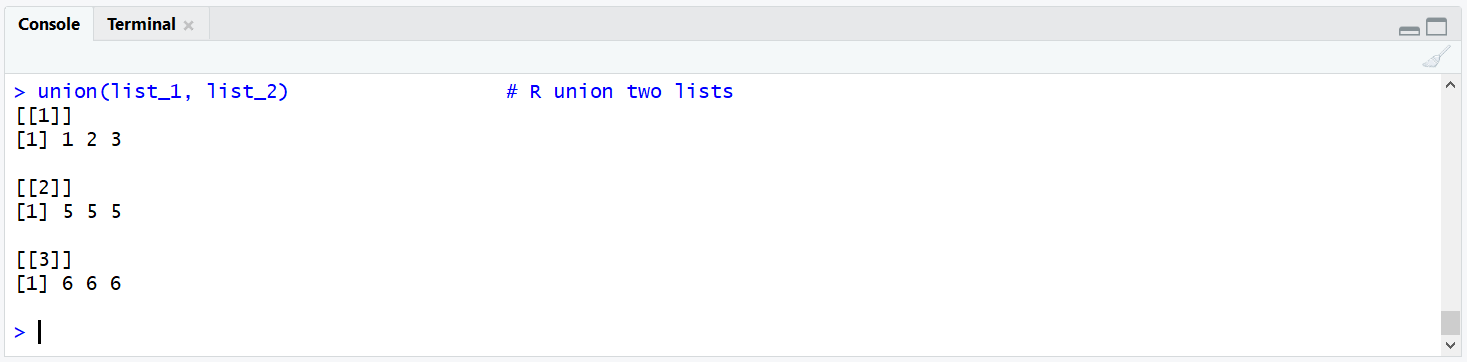# R union Function | 3 Example Codes (Two Vectors, Data Frames & Lists)

Basic R Syntax:

`union(x1, x2)`

In R, the union function returns all values that appear in at least one of two data objects (usually vectors). Each value is returned only once. The basic syntax for the union R command is shown above.

In this article, I’m going to illustrate the usage of the union function on the basis of 3 examples. Let’s start right away…

## Example 1: Union of Two Vectors in R

Let’s begin with two vectors – the simplest way of using union in R. Consider the following two vectors as example:

```x1 <- c(1, 2, 3, 4, 5, 6, 6, 6, 6)    # Example vector 1
x2 <- c(8, 9)                         # Example vector 2```

Keep in mind that the vector X1 consists of the values 1-6; and the vector X2 consists of the values 8-9 (7 is missing). Now, let’s apply the union function:

```union(x1, x2)                         # R union of two vectors
# 1 2 3 4 5 6 8 9```

Beside 7 (the value which didn’t appear in any of the two vectors), the R union function returns all values from 1-9. In other words, the function returns all values that appear at least once either in X1 or X2.

Note: The value 6 is returned only once, even though it appears several times in X1.

In case you need some further explanations on the previous R syntax, you may have a look at the following video of my YouTube channel:

Please accept YouTube cookies to play this video. By accepting you will be accessing content from YouTube, a service provided by an external third party.If you accept this notice, your choice will be saved and the page will refresh.

## Example 2: Union of Two Data Frames

The union command can also be used for the unification of two data frames. Let’s create two data frames first:

```data_x <- data.frame(x1 = c(4, 7, 8), # Data frame 1
x2 = c(5, 5, 5))
# x1 x2
#  4  5
#  7  5
#  8  5

data_y <- data.frame(y1 = c(2, 3, 4), # Data frame 2
y2 = c(5, 5, 5))

# y1 y2
#  2  5
#  3  5
#  4  5```

Note that the second column of the two data tables is equal (i.e. X2 = Y2). Now, let’s apply the union function to these two data frames:

`union(data_x, data_y)                 # R union two data frames`Graphic 1: RStudio Console Output after Applying union() to Two Data Frames.

Graphic 1 shows the output of the RStudio console. The output is a list and each element of the list consists of the values of one column of the two data frames:

• List element 1 consists of the values of the first column of data_x.
• List element 2 consists of the values of the second column of data_x.
• List element 3 consists of the values of the first column of data_y.

The second column of data_y is missing, since it is identical to the second column of data_x (i.e. X2).

If you want to convert the list back to the data.frame format, you can use the following R syntax:

```union_xy <- union(data_x, data_y)     # Store union of data frames
unlist_xy <- unlist(union_xy)         # Unlist union of data frames
data_xy <- data.frame(                # Convert list to data.frame
matrix(unlist_xy, ncol = length(union_xy)))

data_xy
# X1 X2 X3
#  4  5  2
#  7  5  3
#  8  5  4```

## Example 3: Union of List Elements

It is also possible to use the R union function for two lists. Let’s first create two lists in R:

```list_1 <- list()                       # Create example list 1
list_1[] <- c(1, 2, 3)
list_1[] <- c(5, 5, 5)

list_2 <- list()                       # Create example list 2
list_2[] <- c(1, 2, 3)
list_2[] <- c(6, 6, 6)```

Note: The first element of both lists is equal (i.e. list_1[] == list_2[]).

Now, let’s apply the union function to our 2 lists:

`union(list_1, list_2)                  # R union two lists`Graphic 2: RStudio Console Output after Applying union() to Two Lists.

Graphic 2 shows the output of the RStudio console after the application of union to two lists. The output is also a list object that consists of the first two list elements of list_1 as well as of the second list element of list_2. The list element 1 of list_2 is not returned, since the values of this list element are identical with the values of the first element of list_1.

In other words: All unique list elements are returned by the union function.

## Video: union and Other Set Functions

Have a look at the following video of the YouTube channel LearnR for more examples of the R union function and other so called set functions such as intersect, setdiff, or setequal.

Please accept YouTube cookies to play this video. By accepting you will be accessing content from YouTube, a service provided by an external third party.If you accept this notice, your choice will be saved and the page will refresh.